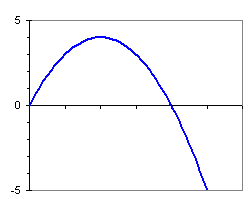From: Jennifer Let's say in you have a fountain and the water jets form parabolic arches. The center of the fountain, being the origin of the coordinate system, it is elevated 5 feet off the ground, . The equation formed the water arch is y= -x2+4x, what is the radius of the basin needed to catch the water at ground level??? Hi Jennifer. The equation of that parabola looks something like this:I've only drawn the portion that really interests us (from the origin to y = -5). The problem asks for the radius of the basin needed to catch the water. That means the horizontal distance from the spout to where it hits ground level. As you can see from the drawing, this is just the positive value of x when y is -5. So put -5 in for the value of y in your equation y = -x2 + 4x and solve for x (you should be able to factor this). You will get two values for x, one of them is your radius!   Hope this helps, Stephen La Rocque.